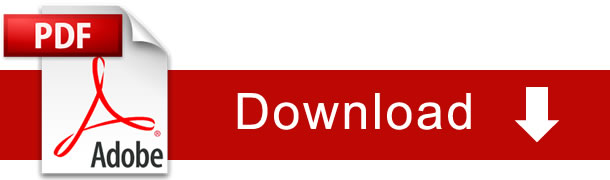Surface Areas And Volumes Notes For Class 10 Chapter 13 Download PDF

## Chapter 13 :- Surface Areas and Volumes

### RIGHT CIRCULAR CYLINDER:

A right circular cylinder is solid generated by the revolution of a rectangle about of its sides.

NOTE : If a paper, cylinder open at both the ends is cut along a vertical line on the curved surface and stretched on a plane surface, we obtain a rectangle of length i.e.,
27πr and breadth= Height of cylinder h.

So, curved surface area (C.S.A) or lateral surface area = 2πr * height Important Formula For Cylinder

1. C. S. A of cylinder = ( Perimeter of base) * Height = 2πrh2. Area of each end of cylinder = 2πr2

3. Total surface area (including both circular ends) = 2πrh + 2πr2 = 27πr(h + r)

4. Volume of cylinder — πr2h = [(Area of base) * height]

Hollow Cylinder’s formulae e.g., (Rubber tubes pipes, etc.)

1. Volume of material = Exterior volume — Interior volume = πR2h — πR2h = πh(R2 – r2)

2. C. S. A or L. S. A = external surface area + internal surface area= 2πRh + 2πrh

3. T. S . A. of hollow cylinder = C. S. A+ 2 ( area of base ring )
= (2πRh + 2πrh) + 2(πR2 – πR2)

##### NOTE:

1. Two end faces of right circular cylinder are circles having each area = πR2
2. Mass of cylinder = Volume * density
3. When rectangular sheet of paper is rolled along its length , we get a cylinder whose base circumference is length of sheet and height is same as breadth of sheet.

### CONE

From figure, AO = height of cone and is denoted by ‘h’OB = radius of the base of cone, AB = slant height of a cone (l) Important Formula Of rt. Circular Cone :

1. Volume of cone = 1 / 3 πR2h

2. C. S. A or L. S. A=πrl where slant height = l =√ R2 + hR2

3. T. S. A of cone = πrl + πR2

#### FRUSTUM OF A CONE

FRUSTUM : A cone is cut by a plane parallel to the base of the cone,Then the portion between the plane and base is called frustum of the cone Important

###### Formulae for Frustum :

1. Volume of frustum of cone = πh / 3[R2 + R2 + Rr] cubic unit
2. L. S. A or C. S. A = πl(R + r) Sq units where l2 = h2 + (R – r)2
3. T. S. A = πR2 + πR2 + πl(R + r) Sq. units. (Area of base + Area of top + Area of lateral )
4. Slant height (l) = √h22 + (R – r)2

##### IMPORTANT FORMULA FOR SPHERE AND HEW-SPHERE

(a) Surface area of sphere = 4πR2(b) Volume of sphere = 4 / 3 πR3
(c) Volume of hemisphere = 2 / 3 πR3
(d) C.S.A. of hemisphere = 2πR2
(e) Total surface area of Hemi-sphere = 2πR2 + πR2 =3πR2

###### IMPORTANT FORMULA FUR SPHERICAL SHELL/ HEMILSPHERICAL SHELL

(a) Outer surface area of spherical shell =4πR2
(b) Inner S.A. of spherical shell = 4πR2
(c) Total surface area of spherical shell = 4π(R2+ R2)
(d) Volume of spherical shell of external radius R and internal radius ‘r’ = 4 / 3π(R3 – R3)
(e) Outer curved surface area hemispherical shell = 2πR2(f) Inner curved surface area of hemispherical shell = 2πR2
(g) Thick hemispherical bowl of external and internal radii R and r, Total S.A. = π(3R2+ R2)

(h) Volume of hemispherical shell of external radius ‘R’ and internal radius ‘r’ = 2 / 3π(R3 — R2).### NCERT Books Free Pdf Download for Class 5, 6, 7, 8, 9, 10 , 11, 12 Hindi and English Medium

 Mathematics Biology Psychology Chemistry English Economics Sociology Hindi Business Studies Geography Science Political Science Statistics Physics Accountancy